• 1、条形图 import seaborn as sb #data指pd的dataframe，x指的坐标，也可以设置为y sb.countplot(data = df, x = 'cat_var') #返回一个 RGB 元组列表，每个元组由三个数字组成，分别对应红绿蓝通道值，这三个值确定...
1、条形图
import seaborn as sb
#data指pd的dataframe，x指的坐标，也可以设置为y
sb.countplot(data = df, x = 'cat_var')
#返回一个 RGB 元组列表，每个元组由三个数字组成，分别对应红绿蓝通道值，这三个值确定一个颜色
sb.color_palette()
#选择第一个
base_color = sb.color_palette()
#指定颜色
sb.countplot(data = df, x = 'cat_var', color = base_color)
#返回降序列表
df['cat_var'].value_counts()
#得到index列表
cat_order = df['cat_var'].value_counts().index
#指定顺序
sb.countplot(data = df, x = 'cat_var', color = base_color, order = cat_order)
#x轴的每一个类别竖向显示
import matplotlib.pyplot as plt
plt.xticks[rotation=90]

在指定条形图每一个条形的排序顺序时，如果是无序分类变量，可以按照高低大小排序，对于有序分类变量，可以按照如下方式
# 本方法要求 pandas 0.21 或更高版本
level_order = ['Alpha', 'Beta', 'Gamma', 'Delta']
ordered_cat = pd.api.types.CategoricalDtype(ordered = True, categories = level_order)
df['cat_var'] = df['cat_var'].astype(ordered_cat)

## 如果你的 pandas 为 0.20.3 或更低版本，请使用这种方法：
# df['cat_var'] = df['cat_var'].astype('category', ordered = True,
#                                      categories = level_order)

base_color = sb.color_palette()
sb.countplot(data = df, x = 'cat_var', color = base_color)

2、条形图相对值
#返回形状，即几行几列的数组shape=rows,shape=columns
dataframe.shape()
#计算最大值站全部的比重
n_points = df.shape
max_count = df['cat_var'].value_counts().max()
max_prop = max_count / n_points
#返回等差列表
tick_props = np.arange(0, max_prop, 0.05)
#转换成%
tick_names = ['{:0.2f}'.format(v) for v in tick_props]
#修改对应轴的值
plt.yticks(tick_props * n_points, tick_names)
#修改对应轴的名称
plt.ylabel('proportion')
#给每一列标注值是多少，并为每个长条添加一个文本元素
for i in range(type_counts.shape):
count=type_counts[i]
pct='{:0.1f}'.format(100*count/n_pokemon)
plt.text(count+1,i,pct,va='center')



展开全文数据可视化
•展开全文• https://zhuanlan.zhihu.com/p/36939840
https://zhuanlan.zhihu.com/p/36939840


展开全文• python 条形图 饼图One of the more popular data visualizations right now is the animated bar chart, in which the bars look like they are racing each other. To create your own bar chart race is ...
python 条形图 饼图One of the more popular data visualizations right now is the animated bar chart, in which the bars look like they are racing each other. To create your own bar chart race is surprisingly easy and does not take long to create.现在，更流行的数据可视化之一是动画条形图，其中的条形看起来像彼此竞赛。 创建自己的条形图竞赛非常容易，而且创建时间并不长。
You will need to install two libraries if you do not already have them. I use homebrew and it makes libraries very easy to install. If you do not already have homebrew, I recommend you install that before continuing. In your terminal, you need to use the code: brew install ffmpeg. This is the software that will encode your data to be used in the animation. 如果您还没有两个库，则需要安装它们。 我使用自制软件，这使得库的安装非常容易。 如果您还没有自制软件，建议您在继续之前进行安装。 在终端中，您需要使用以下代码：brew install ffmpeg。 这是将对要在动画中使用的数据进行编码的软件。
First import all necessary libraries. 首先导入所有必需的库。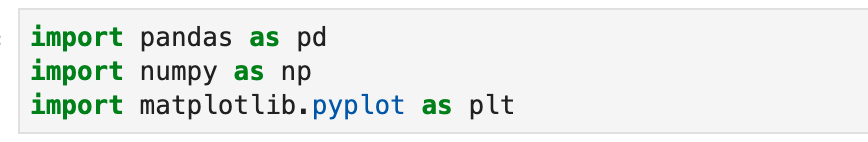Next pip install bar_chart_race. I did this right in my jupyter notebook, but you could also do this in your terminal. 接下来的pip安装bar_chart_race。 我在jupyter笔记本中做到了这一点，但是您也可以在终端中做到这一点。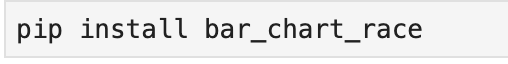In your notebook, import bar_chart_race as bcr 在您的笔记本中，将bar_chart_race导入为bcr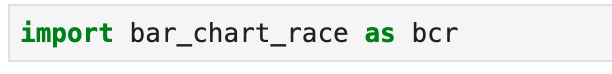bcr has a few examples linked to their github. In this article, I’ll display the Covid-19 deaths. bcr有一些链接到其github的示例。 在本文中，我将展示Covid-19的死亡事件。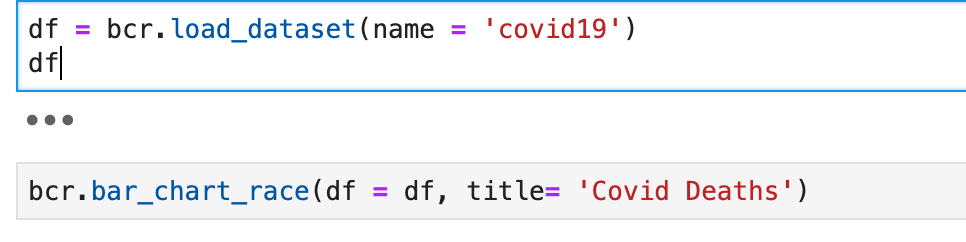The Final product gives us a bar chart race showing the date, number of Covid-19 deaths, and how rapidly each country increases their numbers. 最终产品为我们提供了一个条形图竞赛，显示了日期，Covid-19死亡人数以及每个国家增加其人数的速度。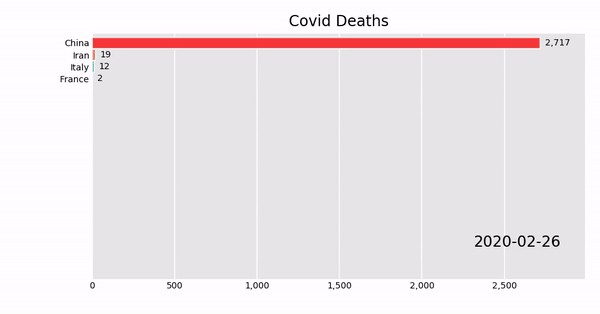You can use your own data from a csv file or excel sheet as long as it has enough information. 只要具有足够的信息，就可以使用csv文件或excel工作表中的数据。
翻译自: https://medium.com/swlh/how-to-create-a-bar-chart-race-python-763856b32181python 条形图 饼图
展开全文• 本文实例为大家分享了python绘制水平条形图的具体代码，供大家参考，具体内容如下水平条形图与绘制柱状图类似，大家可以先看看我之前写的博客，如何绘制柱状图水平条形图需要在Bar函数中设置orientation= 'h'其他的...
• 本文实例为大家分享了python绘制水平条形图的具体代码，供大家参考，具体内容如下水平条形图与绘制柱状图类似，大家可以先看看我之前写的博客，如何绘制柱状图水平条形图需要在Bar函数中设置orientation= 'h'其他的...
• 一、条形图绘制参数详解1、bar(left, height, width=0.8, bottom=None, color=None, edgecolor=None, linewidth=None, tick_label=None, xerr=None, yerr=None, label = None, ecolor=None, align, log=False, **...
• pandas 绘制分组条形图1、 示例 1 使用默认颜色代码import matplotlib.pyplot as pltimport numpy as npimport pandas as pd# 生成数据rng = np.random.RandomState(42)v1...0839相关推荐2019-09-28 21:13 −Python ...
• 我画了3个条形图，问题解决了。在import matplotlib.pyplot as plt;plt.rcdefaults()from numpy.random import randfrom numpy import arangeimport numpy as npdef libra_execution_time():data = np.genfromtxt('C...
• Python-Matplotlib 5 条形图Example 1import numpy as npimport matplotlib.pyplot as pltN = 5y = [20, 10, 30, 25, 15]index = np.arange(5)print(index)#p1 = plt.bar(left=index, height=y)#p1 = plt.bar(left=...
• 条形图是一种常见的图形，那么如何使用python绘制一个条形图呢？我们选用的数据集是kaggle上的bike sharing数据集。数据集的描述如下所示：我们的任务是要观看一下共享单车的使用次数是否和季节有关。我们的方法是...
• 今天python学习了条形图,方便大家参考,所以更新到博客来. # 条形图--->bar # 导入绘图包 import matplotlib.pyplot as plt import numpy as np # 正常显示中文字符 plt.rcParams['font.sans-serif'] = ['SimHei'...可视化 经验分享
• 简单垂直条形图GDP = [12406.8, 13908.57, 9386.87, 9143.64]# 绘图plt.bar(range(4), GDP, align='center', color='steelblue', alpha=0.8)# 添加轴标签plt.ylabel('GDP')# 添加标题plt.title('GDP')# 添加刻度标签...python 条形图
• 今天想看下两组数据的对比情况，就给画了个：classify = ['accept', 'reject'] # 姓名education = list(ac['index'])counts = (list(ac['education']), list(re['education']))# 设置柱形宽度bar_width = 0.35...
• I have a notebook with 2* bar charts, one is winter data & one is summer data. I have counted the total of all the crimes and plotted them in a bar chart, using code:ax = summer["...
• 下面建议的代码生成一个PyQt窗口，其中显示3D条形图。可以使用滑块(时间变化)为图表设置动画。在import sysfrom PyQt4 import QtGui, QtCorefrom matplotlib.backends.backend_qt4agg import FigureCanvasQTA...
• Bar Plots – The king of ...The ability to render a bar plot quickly and easily from data inPandas DataFramesis a key skill for any data scientist working in Python.Nothing beats the bar plot for f...
• 我想画一个堆积条形图。然而，我不能减少间距之间的酒吧。我想保持酒吧狭窄，但酒吧之间没有空间或非常小的空间。在我使用以下代码：#!/usr/bin/env python3# -*- coding: utf-8 -*-"""Created on Mon Aug 21 16:56:...
• 基本上，我正在创建一个条形图，我可以弄清楚如何在条形图上（在条形图的中心或上方）添加值标签。一直在网上查看示例，但在我自己的代码上实现未成功。我相信解决方案是使用“文本”或“注释”，但是我：a）不知道...
• 简介条形图(bar chart)是用宽度相同条形的高度或长短来表示数据多少的图形，可以横置或纵置。纵置时的条形图也称为柱形图(column chart)。绘制条形图1 import matplotlib.pyplot as plot2 3 data = [5., 25., 50., ...python 条形图
• 我正在尝试制作一个负值的条形图，其中基线x轴位于-10而不是0，并且这些值都为-10，因此它们从基线向上延伸。如果按原样绘制，则条形图向下延伸：123import matplotlib.pyplot as pltvals = [-4, -6, -8, -6, -5]plt...
• 简介堆积条形图作为条形图的一种，能够显示单个项目与整体之间的关系。不仅可以直观的看出每个系列的值，还能够反映出系列的总和，尤其是当需要看某一项目的总和以及其组成各系列值的比重时，最适合使用堆积条形图。...python 条形图
• 摘要详细介绍python matpltlib中垂直、水平和堆积三种条形图的绘制。目录1、垂直柱形图 垂直柱形图参数详解 多个垂直柱形图并列显示 柱形图高度显示在柱子上方 堆积柱形图2、水平柱形图‍ ‍‍柱形图参数详解‍‍‍...
• 我创建了一个简单的从0到100%的水平堆积条形图，只需要两个数据就可以知道两者之间的平衡。我希望能够将它们的值写在条内(如果可能的话在中间)。但即使是if this example looks similar，我也找不到一种方法来适应我...
• data.columns[1:]) # 设置Y轴的刻度范围 plt.ylim([0, 500000]) # 为每个条形图添加数值标签 forx_t, y_tinenumerate(data.loc[0, :][1:]): plt.text(x_t, y_t / 2, '%sW'% (round(y_t / 10000, 2)), ha='center', ...
• 这里有一个解决方案，它使用ax.annotate在每个条内绘制箭头。由于OP不太清楚箭头应该是什么样子，所以我把每个条分成矩形(在代码中我称它们为squares，但如果你确定了绘图的纵横比，它们就只是正方形)，并为每个矩形......

# python条形图python 订阅